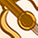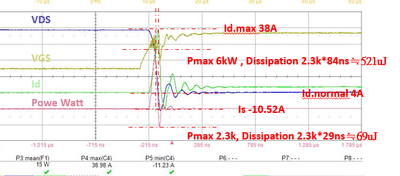Announcements

# MOSFET (Si/SiC) Forum DiscussionsLevel 3Level 3

# Eon loss with Temperature Question

Dear Sir,

How to evaluate power device junction temperature, at the time of Eon?
For example, the power dissipation I see is about 6kW+2.3kW,
Is my temperature rise equal to TA + (6kW+2.3kW) *RJA?1 SolutionModeratorModerator

# Re: Eon loss with Temperature Question

Hello Kuowenyung,

Temperature rise has to be estimated based on the average losses and not considering the peak dissipation.

You may estimate the average loss during turn-on as below, by approximating the power curve as a triangle.

Psw_on = (1/2 * ton * Vds*Id)/T

where T - total switching period

Here 6kW would account for Vds*Id.

Temperature rise due to the turn-on losses  can be calculated as below

Tj= Psw_on * Rthj-a + Ta

But you may not be able to observe this change in real-time as temperature rise will be gradual and not instantaneous.

Hence to estimate the actual junction temperature of your device, it is advised to estimate the total power loss over a switching period, which will include both conduction and switching losses and then calculate the junction temperature as below.

Tj= Ploss*Rthj-a + Ta

where Ploss = Pcond + Psw

I hope this helps. Please feel free to reach out to us if you need further clarification on this topic.

Regards

Meghana RModeratorModerator

# Re: Eon loss with Temperature Question

Hello Kuowenyung,

Temperature rise has to be estimated based on the average losses and not considering the peak dissipation.

You may estimate the average loss during turn-on as below, by approximating the power curve as a triangle.

Psw_on = (1/2 * ton * Vds*Id)/T

where T - total switching period

Here 6kW would account for Vds*Id.

Temperature rise due to the turn-on losses  can be calculated as below

Tj= Psw_on * Rthj-a + Ta

But you may not be able to observe this change in real-time as temperature rise will be gradual and not instantaneous.

Hence to estimate the actual junction temperature of your device, it is advised to estimate the total power loss over a switching period, which will include both conduction and switching losses and then calculate the junction temperature as below.

Tj= Ploss*Rthj-a + Ta

where Ploss = Pcond + Psw

I hope this helps. Please feel free to reach out to us if you need further clarification on this topic.

Regards

Meghana R